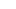# SSC CGL 2020 Syllabus, Preparation Tips and Strategy for Quantitative AptitudeAccording to the latest notification the SSC will conduct Tier – I CGL Exam from 2nd March to 11th March 2020 and Tier-II CGL Exam from 22nd June 2020 to 25th June 2020 in online mode.

EXAMINATION PATTERN OF QUANTITATIVE APTITUDE

In the section of Quantitative Aptitude of SSC CGL Tier-1 Exam, there will be 25 multiple-choice questions and each question carries 2 marks. However, there will be negative marking of I 0.50 marks for each wrong answer

In SSC CGL Tier-II Exam the Paper I – Quantitative Aptitude consists of 100 multiple-choice questions, and each question carries 2 marks. The duration of the examination is 2 hours and there will be a negative marking of 0.50 marks for each wrong answer

DETAILED CHAPTER-WISE SYLLABUS OF QUANTITATIVE APTITUDE SECTION

1. Arithmetic

The chapters under Arithmetic are:

• Number System
• Fractions and Decimals
• Square roots
• Percentage
• Profit, Loss and Discount
• Ratio & Proportion
• Average
• Time and Distance
• Time and Work
• Pipes and Cisterns
• Mixture and Alligation
• Simple Interest & Compound Interest
• Probability
• Permutation and Combination
1. Algebra
• Basic algebraic identities of School Algebra & Elementary surds
• Graphs of Linear Equations

Stay connected with fellow students on PaGaLGuY for SSC CGL 2019 Tier 1- Syllabus and Preparation

1. Geometry
• Triangle and its various kinds of centers
• Congruence and similarity of triangles
• Regular Polygon
• Right Prism & Hemispheres
• Circle and its chords, tangents
• Angles subtended by chords of a circle
• Common tangents to two or more circles
• Right Circular Cone
• Sphere
• Cylinder
• Right Circular Cylinder
• Regular Right Pyramid with Triangular base or Square base
• Rectangular Parallelepiped
1. Mensuration
• Two-dimensional (2D) and Three-dimensional (3D) Mensuration
1. Trigonometry
• Trigonometric Ratios
• Complementary Angles
• Standard Identities
• Height and Distance

PREPARATION TIPS AND STRATEGIES

• While preparing for the Quantitative Aptitude Section – SSC CGL Exam 2019-20, an important thing to remember by the candidate is that they should work on their basics and they should try to focus on the basics of each of the topics in order to gain the in-depth knowledge about the topics.
• The next thing to consider is that the students will have to manage the time properly. The candidates will have to spend more time on the weaker areas. Doing this, the candidates can allocate less time for the strength areas so that they can give equal importance to each topic
• Also, they have to practice previous year papers as much as they can so that they can get an idea of the current structure of the examination. Practising the previous year papers is important as the exam pattern keeps on changing
• In order to prepare well for the examination, the candidates will have to prepare a proper strategy and a time table so that they can work according to the routine for better results.
1 comment
1. @xabhi007
Watch these videos to revise your concepts https://youtu.be/B1DacxVJdlA
January 2020